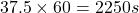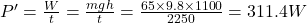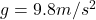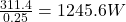## The record time for a Tour de France cyclist to ascend the 1100-mm-high Alpe d’Huez is 37.5 minmin. The rider and his bike had a mass of 65

Question

The record time for a Tour de France cyclist to ascend the 1100-mm-high Alpe d’Huez is 37.5 minmin. The rider and his bike had a mass of 65 kg. At the average speed of the ride, the metabolic power to ride on level ground would be 700 W. To this, we must add the power to make the climb. What was his total metabolic power?

in progress 0
2 months 2021-07-30T09:53:44+00:00 1 Answers 1 views 0

1945.6 W

Explanation:

We are given that

Height,h=1100 m

Time,t=37.5 min=1 min=60 s

Mass,m=65 kg

Power,P=700 W

We have to find the his total metabolic power.

Power,=WhereEfficiency =25%

Therefore,Power,P’=Total metabolic power=P+P’=700+1245.6=1945.6 W